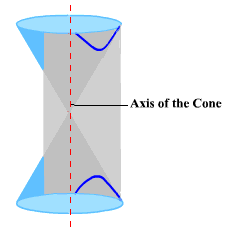# More on hyperbolas

A hyperbola is the set of all points $P$ in the plane such that the difference between the distances from $P$ to two fixed points is a given constant.  Each of the fixed points is a focus .  (The plural is foci.)  If $P$ is a point on the hyperbola and the foci are ${F}_{1}$ and ${F}_{2}$ then $\stackrel{¯}{P{F}_{1}}$ and $\stackrel{¯}{P{F}_{2}}$ are the focal radii .  As you can see, the graph of the hyperbola has two disconnected branches that look similar to parabolas.  Each branch approaches diagonal asymptotes .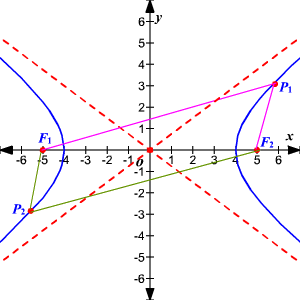The center of a hyperbola is the midpoint of the line segment joining its foci.  The transverse axis is the line segment that contains the center of the hyperbola and whose endpoints are the two vertices of the hyperbola.

A central hyperbola, one with its center on the origin and its foci on either the $x$ -axis or $y$ -axis has one to the two formulas below.  Note that ${a}^{2}$ is the denominator of the positive term in each case.

The hyperbola with its center at $\left(0,0\right)$ , foci $\left(c,0\right)$ and $\left(-c,0\right)$ and distance between the vertices $2a$ has the equation

$\frac{{x}^{2}}{{a}^{2}}-\frac{{y}^{2}}{{b}^{2}}=1$ where ${b}^{2}={c}^{2}-{a}^{2}$ .

The equations of the asymptotes are $y=\frac{b}{a}x$ and $y=-\frac{b}{a}x$

Note that the hyperbola will have either $x$ - or $y$ -intercepts but never both.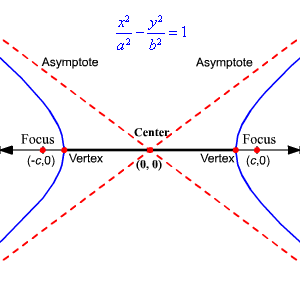The hyperbola with its center at $\left(0,0\right)$ , foci $\left(0,c\right)$ and $\left(0,-c\right)$ , then the equation is $\frac{{y}^{2}}{{a}^{2}}-\frac{{x}^{2}}{{b}^{2}}=1$ with ${b}^{2}={c}^{2}-{a}^{2}$

The equations of the asymptotes are $y=\frac{a}{b}x$ and $y=-\frac{a}{b}x$ .

Example 1:

Find an equation of the hyperbola having foci $\left(3,0\right)$ and $\left(-3,0\right)$ and the distance between the vertices equal to $4$ if its center is at the origin.

The distance from each focus to the center is $3$ , so $c=3$

The distance between the vertices is $2a$ .  So, $2a=4$ and $a=2$ .

${b}^{2}={c}^{2}-{a}^{2}$ , so ${b}^{2}={3}^{2}-{2}^{2}=9-4=5$

Since the foci are on the $x$ -axis, the equation is $\frac{{x}^{2}}{4}-\frac{{y}^{2}}{5}=1$ .

Example 2:

For the above example, find the equations of its asymptotes and graph the hyperbola.

The equations of the asymptotes are $y=\frac{\sqrt{5}}{2}x$ and $y=-\frac{\sqrt{5}}{2}x$ .

The $x$ -intercepts are $\left(2,0\right)$ and $\left(-2,0\right)$ .  There are no $y$ -intercepts.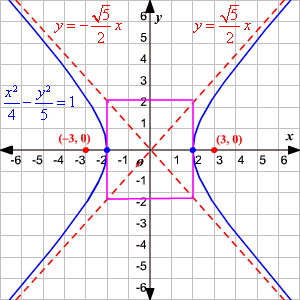The graph of a hyperbola can be translated so that its center is at the point $\left(h,k\right)$ .  This means that the graph has been translated $h$ units on the horizontal axis and $k$ units on the vertical axis.

Horizontal Major Axis Vertical Major Axis

$\frac{{\left(x-h\right)}^{2}}{{a}^{2}}-\frac{{\left(y-k\right)}^{2}}{{b}^{2}}=1$    $\frac{{\left(y-k\right)}^{2}}{{a}^{2}}-\frac{{\left(x-h\right)}^{2}}{{b}^{2}}=1$

Foci at $\left(h-c,k\right)$ and $\left(h+c,k\right)$ Foci at $\left(h,k-c\right)$ and $\left(h,k+c\right)$

Example 3:

Find an equation of the hyperbola with foci $\left(-3,-2\right)$ and $\left(-3,8\right)$ and the distance between the vertices $8$ .

The distance between the vertices, $2a$ , is $8$ .  So, $2a=8$ and $a=4$ .

The center is halfway between the foci at $\left(-3,3\right)$ .

The distance from the center to each focus is $5$ so $c=5$ .

${b}^{2}={c}^{2}-{a}^{2}={5}^{2}-{4}^{2}=25-16=9$

Therefore, the equation of the hyperbola is $\frac{{\left(y-3\right)}^{2}}{16}-\frac{{\left(x+3\right)}^{2}}{9}=1$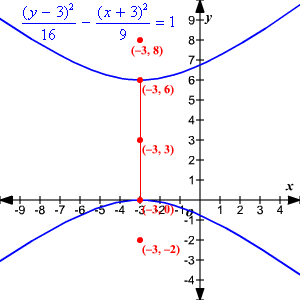A hyperbola can also be defined as a conic section obtained by the intersection of a double cone with a plane that is intersects both pieces of the cone without intersecting the axis.